# Short Answer Questions: Polynomials - 2 Notes | Study Mathematics (Maths) Class 10 - Class 10

## Class 10: Short Answer Questions: Polynomials - 2 Notes | Study Mathematics (Maths) Class 10 - Class 10

The document Short Answer Questions: Polynomials - 2 Notes | Study Mathematics (Maths) Class 10 - Class 10 is a part of the Class 10 Course Mathematics (Maths) Class 10.
All you need of Class 10 at this link: Class 10

Ques 21: Find the zeroes of the quadratic polynomial 5x2 - 4 - 8x and verify the relationship between the zeroes and the coefficients of the polynomial.
Sol:
p (x) = 5x2 - 4 - 8x
= 5x2 - 8x - 4
= 5x2 - 10x + 2x - 4
= 5x (x - 2) + 2 (x - 2)
= (x - 2) (5x + 2)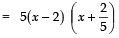∴ zeroes of p (x) are 2 and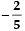Relationship Verification
Sum of the zeroes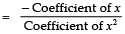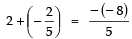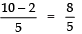⇒ 8/5 = 8/5

i.e., L.H.S. = R.H.S. ⇒ relationship is verified.
Product of the zeroes =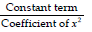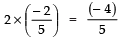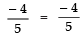i.e., L.H.S. = R.H.S.
⇒ The relationship is verified.

Ques 22: Find the quadratic polynomial, the sum of whose zeroes is 8 and their product is 12. Hence, find the zeroes of the polynomial.
Sol:
∵ The quadratic polynomial p (x) is given by
x2 - (Sum of the zeroes) x + (Product of the zeroes)
∴ The required polynomial is
= x2 -  x + 
= x2 - 8x + 12
To find zeroes:
∵ x2 - 8x + 12 = x2 - 6x - 2x + 12
= x (x - 6) - 2 (x - 6)
= (x - 6) (x - 2)
∴ The zeroes of p (x) are 6 and 2.

Ques 23: If one zero of the polynomial (a2 - 9) x2 + 13x + 6a is reciprocal of the other, find the value of ‘a’.
Sol:
Here, p (x) = (a2 - 9) x2 + 13x + 6a
Comparing it with Ax2 + Bx + C, we have:
A = (a2 - 9); B = 13; C = 6
Let one of the zeroes = a
∴ The other zero = 1/α
Now, Product of the zeroes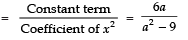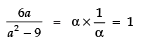⇒ 6a = a2 − 9    ⇒ a2 − 6a + 9 = 0
⇒ (a − 3)2 =0   ⇒ a − 3=0
⇒ a = 3
Thus, the required value of a is 3.

Ques 24: If the product of zeroes of the polynomial ax2 - 6x - 6 is 4, find the value of ‘a’
Sol: Here, p (x) = ax2 - 6x - 6
∵ Product of zeroes =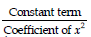but product of zeroes is given as 4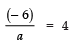⇒ − 6 = 4 × a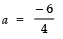⇒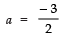Thus, the required value of a is -3/2.

Ques 25: Find all the zeroes of the polynomial x4 + x3 - 34x2 - 4x + 120, if two of its zeroes are 2 and - 2.
Sol:
Here p (x) = x4 + x3 - 34x2 - 4x + 120
∵ The two zeroes of p (x) are 2 and - 2
∴ (x - 2) and (x + 2) are factors of p (x)
⇒ (x - 2) (x + 2) is a factor of p (x)
⇒ x2 - 4 is a factor of p (x).
Now, dividing p(x) by x2 - 4, we have: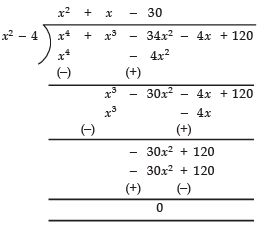∵ Remainder = 0
∴ p (x) = (x2 - 4) (x2 + x - 30)
i.e., x2 + x - 30 is also a factor of p (x).
∵ x2 + x - 30 = x2 + 6x - 5x - 30 = x (x + 6) - 5 (x + 6)
= (x + 6) (x - 5) = [x - (- 6)] [x - 5]
- 6 and 5 are also zeroes of p (x).
⇒ All the zeroes of the given polynomial are : 2, - 2, 5 and - 6

Ques 26: Find all the zeroes of the polynomial 2x4 + 7x3 - 19x- 14x + 30, if two of its zeroes are √2 and -√2.
Sol: P(x) = 2x4 + 7x3 - 19x2 - 14x + 30
∵ √2 and -√2 are the two zeroes of p (x).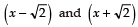are the factors of p (x).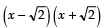i.e., x2 - 2 is a factor of p (x).
Now, dividing p (x) by x2 - 2, we have: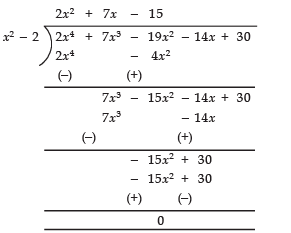∴ p (x) = (2x2 + 7x - 15) (x2 - 2)
[∵ Remainder = 0]
⇒ 2x2 + 7x - 15 is a factor of p (x)
∵ 2x2 + 7x - 15 = 2x2 + 10x - 3x - 15
= 2x (x + 5) - 3 (x + 5)
= (2x - 3) (x + 5)
=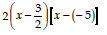∴ 3/2 and - 5 are zeroes of p (x)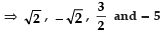are the zeroes of p (x).

Ques 27: Find the quadratic polynomial whose zeroes are 1 and - 3. Verify the relation between the coefficients and the zeroes of the polynomial.
Sol:
∵ The given zeroes are 1 and - 3.
∴ Sum of the zeroes = 1 + (- 3) = - 2
Product of the zeroes = 1 × (- 3) = - 3
A quadratic polynomial p (x) is given by
x2 - (sum of the zeroes) x + (product of the zeroes)
∴ The required polynomial is
x- (- 2) x + (- 3)
⇒ x2 + 2x - 3
Verification of relationship
∵ Sum of the zeroes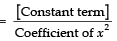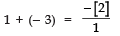⇒− 2= − 2

i.e., L.H.S = R.H.S ⇒ The sum of zeroes is verified
∵ Product of the zeroes =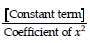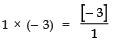⇒− 3= − 3

i.e., L.H.S = R.H.S ⇒ The product of zeroes is verified.

Ques 28: Find the zeroes of the quadratic polynomial 4x2 - 4x - 3 and verify the relation between the zeroes and its coefficients.
Sol: Here, p (x) = 4x2 - 4x - 3 = 4x2 - 6x + 2x - 3
= 2x (2x - 3) + 1 (2x - 3)
= (2x - 3) (2x + 1)
=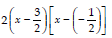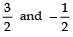are zeroes of p (x).

Verification of relationship
∵ Sum of the zeroes =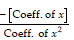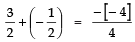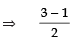⇒ 2/2 = 1 ⇒ 1= 1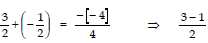2/2 = 1 ⇒ 1 = 1
i.e., L.H.S = R.H.S ⇒ Sum of zeroes is verified
Now, Product of zeroes =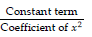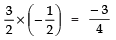⇒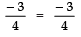i.e., L.H.S = R.H.S ⇒ Product of zeroes is verified.

Ques 29: Obtain all other zeroes of the polynomial 2x3 - 4x - x2 + 2, if two of its zeroes are √2 and -√2.
Sol: p (x) = 2x- 4x - x2 + 2
√2 and -√2 are the zeroes of p (x)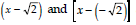and are the factors of p (x)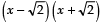is a factor of p (x)
⇒ x2 - 2 is a factor of p (x)
Now, Dividing p (x) by (x2 - 2), we have: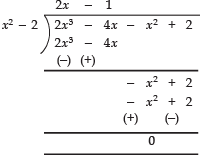⇒ p (x) = (x2 - 2) (2x - 1)
∴ 2x - 1 is also a factor of p (x)
i.e.,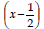is another factor of px.
⇒ 1/2 is another zero of p (x)

Ques 30: Find all the zeroes of x4 - 3x3 + 6x - 4, if two of its zeroes are √2 and - √2.
Sol:
p (x) = x- 3x3 + 6x - 4
2 and (- √2) are the zeroes of p(x)
∴ x-2 and x-(- 2) are factors of p (x)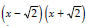is a factor of p (x).
⇒ x2 - 2 is a factor of p (x)
On Dividing p (x) by x- 2, we have: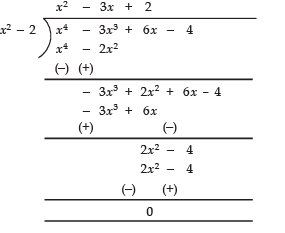Since, remainder = 0
∴ (x2 - 2) (x2 - 3x + 2) = p (x)
Now, x2 - 3x + 2 = x2 - 2x - x + 2
= x (x - 2) - 1 (x - 2) = (x - 1) (x - 2)
i.e., (x - 1) (x - 2) is a factor of p (x)
∴ 1 and 2 are zeroes of p (x).
∴ All the zeroes of p (x) are ,2 , - √2, 1 and 2.

Ques 31: Find a quadratic polynomial whose zeroes are - 4 and 3 and verify the relationship between the zeroes and the coefficients.
Sol:
We know that:
P (x) = x2 - [Sum of the zeroes] x + [Product of the zeroes] ...(1)
∵ The given zeroes are - 4 and 3
∴ Sum of the zeroes = (- 4) + 3 = - 1
Product of the zeroes = (- 4) × 3 = - 12
From (1), we have
x2 - (- 1) x + (- 12)
= x2 + x - 12    ...(2)
Comparing (2) with ax2 + bx + c, we have
a = 1, b = 1, c = - 12
∴ Sum of the zeroes = -b/a
⇒ (+ 3) + (- 4) = -1/1
i.e., L.H.S = R.H.S ⇒ Sum of zeroes is verified.
Product of zeroes = c/a
⇒ 3 × (- 4) = -12/1
⇒ - 12 = - 12
i.e., L.H.S = R.H.S ⇒ Product of roots is verified.

Ques 32: Using division algorithm, find the quotient and remainder on dividing f (x) by g (x), where f (x) = 6x3 + 13x2 + x - 2 and g (x) = 2x + 1
Sol:
Here, f (x) = 6x3 + 13x2 + x - 2
g (x) = 2x + 1
Now, dividing f (x) by g (x), we have: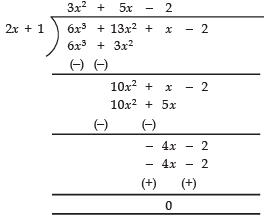Thus, The quotient = 3x2 + 5x - 2
remainder = 0

Ques 33: If the polynomial 6x4 + 8x3 + 17x2 + 21x + 7 is divided by another polynomial 3x2 + 4x + 1 then the remainder comes out to be ax + b, find ‘a’ and ‘b’.
Sol:
We have: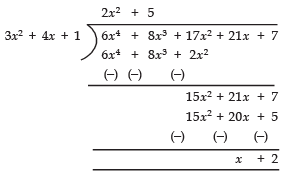∴ Remainder = x + 2
Comparing x + 2 with ax + b, we have
a = 1 and b = 2
Thus, the required value of a = 1 and b = 2.

Ques 34: If the polynomial x4 + 2x3 + 8x2 + 12x + 18 is divided by another polynomial x2 + 5, the remainder comes out to be px + q. Find the values of p and q.
Sol:
We have: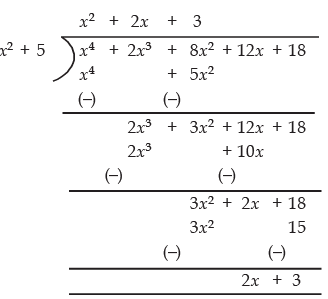∴ Remainder = 2x + 3
Comparing 2x + 3 with px + q, we have
p = 2 and q = 3

Ques 35: Find all the zeroes of the polynomial x3 + 3x2 - 2x - 6, if two of its zeroes are - √2 and √2.
Sol:
p (x) = x3 + 3x2 - 2x - 6
∵ Two of its zeroes are -√2 and √2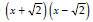is a factor of p (x)
⇒ x2 - 2 is a factor of p (x).
Now, dividing p (x) by x2 - 2 we have: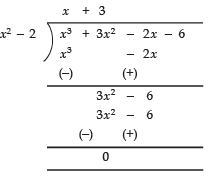∴ p (x) = (x2 - 2) (x + 3)
i.e., (x + 3) is a factor of p (x),
⇒ (- 3) is a zero of p (x)
∴All the zeroes of p (x) are - √2, √2 and - 3.

Ques 36: Find all the zeroes of the polynomial 2x3 + x2 - 6x - 3, if two of its zeroes are -√3 and √3.
Sol:
p (x) = 2x3 + x2 - 6x - 3
Two of its zeroes are -√3 and √3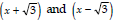and are factors of p (x)
i.e.,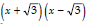is a factor of p (x)
⇒ x2 - 3 is a factor of p (x)
Now, Dividing p (x) by x2 - 3, we have: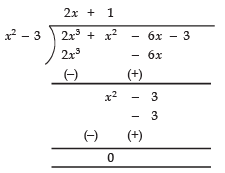∴ p (x) = (x2 - 3) (2x + 1)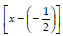is a factor of p (x)
⇒ -1/2 is a zero of p (x)
∴ All the zeroes of p (x) are -√3 , √3 and -1/2.

Ques 37: Find the zeroes of the polynomial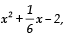and verify the relation between the coefficients and the zeroes of the above polynomial.
Sol: The given polynomial is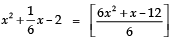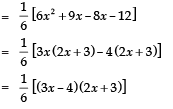∴ zeroes of the given polynomial are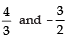Now in,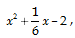co-efficient of x2 = 1
co-efficient of x = 1/6
constant term = –2
∴ Sum of zeroes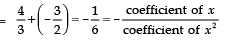Product of zeroes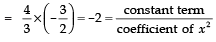Ques 38: Find the quadratic polynomial, the sum and product of whose zeroes are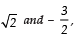respectively. Also find its zeroes.
Sol: Sum of zeroes = √2
Product of zeroes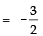∵ A quadratic polynomial is given by
x2 – [sum of roots] x + [Product of roots]
∴ The required polynomial is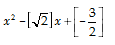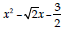Since =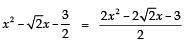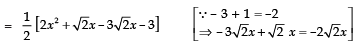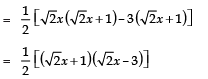⇒ zeroes are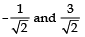Ques 39: If the remainder on division of x3 + 2x2 + kx + 3 by x - 3 is 21, then find  the quotient and the value of k. Hence, find the zeroes of the cubic polynomial x3 + 2x2 + kx - 18.
Sol: Let x3 + 2x2 + kx + 3 = p(x)
∵ The divisor = x – 3
∴ p(3) = 33 + 2 × 32 + 3k + 3
21 = 27 + 18 + 3k + 3
[∵ Remainder = 21]
⇒ 21 – 18 – 3 – 27 = 3k
⇒ –27 = 3k ⇒ k = – 9
Now, the given cubic polynomial
= x3 + 2x2 – 9x + 3
since,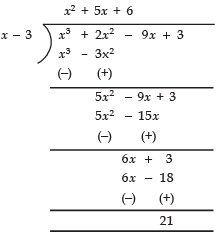∴ The required quotient = x2 + 5x + 6
Now, x3 + 2x2 – 9x – 18 = (x – 3) (x2 + 5x + 6)
= (x – 3) (x + 3) (x + 2)
⇒ The zeroes of x3 + 2x2 – 9x – 18 are 3, –3 and – 2

Ques 40: If a and b are zeroes of the quadratic polynomial x2 – 6x + a; find the value of ‘a’ if 3α + 2β = 20.
Sol:
We have quadratic polynomial = x2 – 6x + a ...(1)
∵ a and b are zeroes of (1)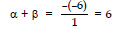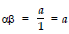It is given that: 3α + 2β = 20      ...(2)
Now, α +β = 6    ⇒ 2 (α+ β) = 2(6)
2α + 2β = 12      ...(3)
Subtracting (3) from (2), we have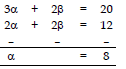Substituting a = 8 in α + β= 6, we get
8 +β = 6 ⇒ β = –2
Since, αβ = a
8(–2) = α ⇒ α = –16

The document Short Answer Questions: Polynomials - 2 Notes | Study Mathematics (Maths) Class 10 - Class 10 is a part of the Class 10 Course Mathematics (Maths) Class 10.
All you need of Class 10 at this link: Class 10Use Code STAYHOME200 and get INR 200 additional OFF

## Mathematics (Maths) Class 10

58 videos|354 docs|138 tests

Track your progress, build streaks, highlight & save important lessons and more!

,

,

,

,

,

,

,

,

,

,

,

,

,

,

,

,

,

,

,

,

,

;